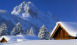# Smekta™

Smekta™ is a text analysing system to allow user (webmaster) prepare data and then use it within html scope.

## Simple variables

Let's define a variable foo:

``````<?php
\$foo = 'bar';
?> ``````

And then within a html contents you can use it:

``<div>My name is <b>{foo}</b></div>``

Such expression will result:

My name is bar

## Arrays and objects

You can access arrays and objects using dots, for example:

``````<?php
?>``````

will be accessed:

`{address.city}`

Both arrays and objects can be nested, e.g.:

``````<?php
\$config=array();
\$config['cache']['timeout'] = 3600;
?>``````

will be accessed:

``{config.cache.timeout}``

## Conditions

If you require conditions you need to declare or not declare (if you want to use negative condition) in php scope. Then use if and endif expressions

``````<?php
\$winter = true;
\$summer = false;
?>``````

And then in html

``````<div>
So, we have
{if:summer}summer <img src="summer.jpg" alt="summer"/>{endif:summer}
{if:winter}winter <img src="winter.jpg" alt="winter"/>{endif:winter}
{if:spring}spring <img src="spring.jpg" alt="spring"/>{endif:spring}
{if:fall}fall <img src="fall.jpg" alt="fall"/>{endif:fall}
</div>
``````

So, we have winter### Negative conditions

You can always want to use negative condition addig ! sign to the expression. Important: you have to use the same negation sign to endif expression:

``````{if:!summer}We are not happy :-({endif:!summer}
``````

### Conditions with comparing

Conditions described above assume only true or false values. If you want to compare a variable to a constant you should use = sign:

``````{if:season=fall}Leaves are falling{endif:season=fall}
``````

remember also to add the = sign to endif expression.

Of course it also works with negative comparition:

``````{if:!season=summer}Children go to school{endif:!season=summer}
``````

You can also compare to more strings in OR convension

``````{if:season=(spring,summer)}I love {season}{endif:season=(spring,summer)}
``````

### Comparing to variables

You don't always want to compare variables to constatnts. Sometimes there is a need to compare variable to another variable

``````<?php
\$foo = 'bar';
\$a = 'bar';
?> ``````

In HTML use:

``{if:foo=\$a}My name is {foo}.{endif:foo=\$a} ``

### Default variables

If you don't know if a variable is defined then you can tell Smekta to use default value.

``````We have beatyfull {season?summer} this year.
``````

Obviously if both variable and default value are not defined, the output will result the oryginal expression. So, if \$season is not defined and you want to avoid displaying {season} in yout HTML output, put the question mark with empty default value

``````<input type="text" name="season" value="{season?}"/>
``````

### Scopes

If your variable set is big and you want to have it ordered and devide it to array indexes, you can use with expression

``````<?php
\$config=array();
\$config['cache']['timeout'] = 3600;
\$config['page']['title'] = 'webkameleon';
?>``````

now you can access timeout variable as described in section Arrays and objects or

``````{with:config}
Timeout={cache.timeout}
{endwith:config}``````

### Loops

If you want to use loops in HTML scope you have to prepare an array with elements witch are also arrays indexed by field named

``````<?
\$people = array(
array('firstname'=>'John', 'lastname'=>'Smith'),
array('firstname'=>'Julia', 'lastname'=>'Clark'),
);
?>``````

Present in HTML:

``````<table>
<tr>
<th>First name</th>
<th>Last name</th>
</tr>
{loop:people}
<tr>
<td>{firstname}</td>
<td>{lastname}</td>
</tr>
{endloop:people}
</table>``````

#### Simple loops

Assume we have a simple array and you want to present its data

``````<?php
?>``````

Can be used as (key,value) representation. The expression {__loop__} represents the key and {loop} represents its value.

``````<h1>Data</h1>
<ul>
<li>{__loop__}: {loop}</li>
</ul>
``````

#### Special variables for loops

There are some predefined variables inside (or outside) loops user can find useful:

• {__index__}: iterating index starting from 1
• {__count__arrayName}: number of items in the array arrayName
• {first}: true if it is first element of the loop array
• {last}: true if it is last element of the loop array

#### Including files

If you have a HTML contents that is going to be multiplicated in the same code or diffrent codes, it is considerable to put it in other file and use {include:} expression

``````<html>
<body>
...
</body>
</html>
``````

#### Functions and operators

User may want to use simple PHP functions to calculate ultimate values of variables used. To call a function, you shoul use | sign. The finction (if exists) will be called assuming the variable to be its first argument, e.g

``````My name is {name} (length of my name is {name|strlen})
``````

Sometimes you would like to use more arguments, for example fisplay only 10 first characters of your name

``````Name: {name|substr:0,10}
``````

First argument of the function substr is the variable (name), second and third are placed after : sign.

There are also possiblity to use simple operators like: +, -, *, / and %, for example

``````<div class="{if:__index__|%:2}odd{endif:__index__|%:2}">
``````

#### Debug

User can use a debug feature in the Smekta™ scope. Using an expression {debug} will display clickable # sign presenting variable set in the data scope. If you put {debug} inside the {loop:} or {with:} expressions the result will differ from the one put at the beginning of HTML file. When you know the name of variable, you can use {debug.nameOfVariable} expression to display its contents. Note that {debug} expressions are not nested, to only first level of variables is usable.